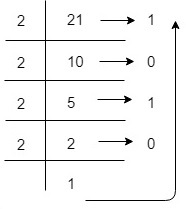# Binary Number Systemn

Binary Number System is one the type of Number Representation techniques. It is most popular and used in digital systems. Binary system is used for representing binary quantities which can be represented by any device that has only two operating states or possible conditions. For example, a switch has only two states: open or close.

In the Binary System, there are only two symbols or possible digit values, i.e., 0 and 1. Represented by any device that only 2 operating states or possible conditions. Binary numbers are indicated by the addition of either an 0b prefix or an 2 suffix.

Position of every digit has a weight which is a power of 2. Each position in the Binary system is 2 times more significant than the previous position, that means numeric value of a Binary number is determined by multiplying each digit of the number by the value of the position in which the digit appears and then adding the products. So, it is also a positional (or weighted) number system.

Most Significant Bit (MSB)Binary PointLeast Significant Bit (LSB)
2221202-12-22-3
4210.50.250.125

Voltage range between 0V to 0.8V is used for binary logic 0 and voltage range between 2V to 5V is used for binary logic 1. Voltage range between 0.8V to 2V is not used because it may causes error in a digital circuit.

Example-1 − The number 125 is interpreted as

125 = 1x26+1x25+1x24+1x23+1x22+0x21+1x20=1111101

Here, right most bit 1 is the least significant bit (LSB) and left most bit 1 is the most significant bit (MSB).

Example-2 − The number 90.75 is interpreted as

90.75 = 1x26+0x25+1x24+1x23+0x22+1x21+0x20+1x2-1+1x2-2=1011010.11

Here, right most bit 1 is the least significant bit (LSB) and left most bit 1 is the most significant bit (MSB).

Example-3 − A decimal number 21 to represent in Binary representation(21)10 =16+0+4+0+1 = 1x24+0x23+1x22+0x21+1x20 =(10101)2
So, decimal value 21 is equivalent to 10101 in Binary Number System.

## Applications of Binary Number System

The binary number system is very useful in computer technology and computer programming languages also uses binary number system that is helpful in digital encoding. The binary number system can also be used in Boolean algebra.